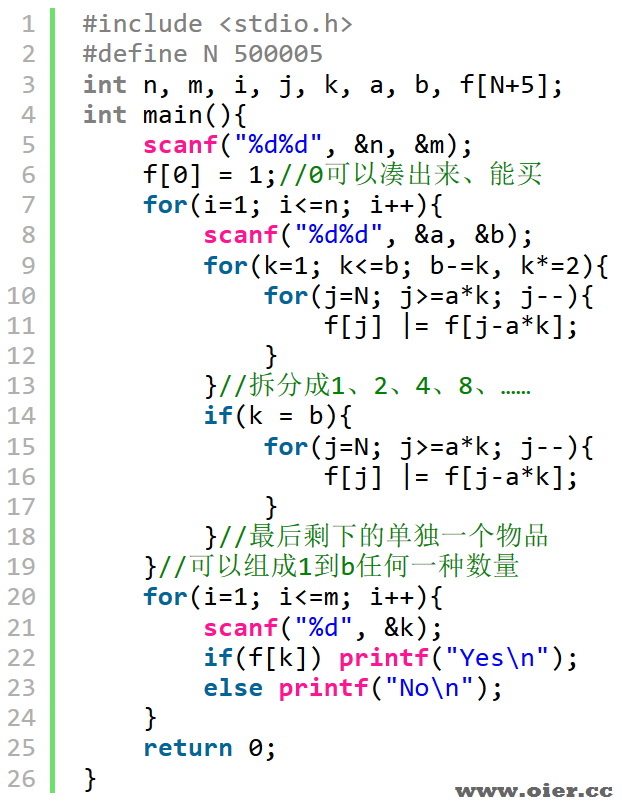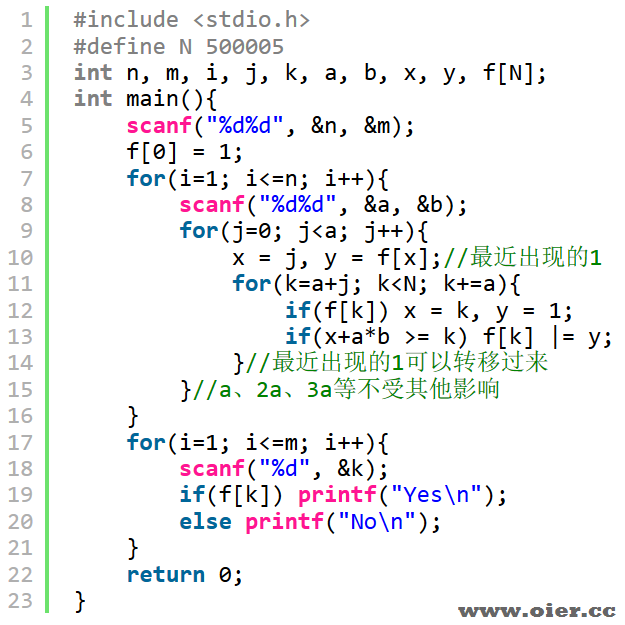580+

## 题目描述

Jimmy 到 Symbol 的手表店买手表，Jimmy 只带了 $n$ 种钱币，第 $i$ 种钱币的面额为 $k_i$ 元，张数为 $a_i$ 张。Symbol 的店里一共有 $m$ 块手表，第 $i$ 块手表的价格为 $t_i$ 元。

Symbol 的手表店不能找零，所以 Jimmy 只能在凑出恰好的钱数时才能购买一块手表。现在对于店里的每块手表，Jimmy 想知道他能不能凑出恰好的钱数进行购买。

## 输入输出样例

### 输入样例 #1

3 5
1 2
5 1
6 3
3 19 21 1 7

### 输出样例 #1

No
Yes
No
Yes
Yes


## 说明

– 第二块手表 $19=6 \times 3+1=6 \times 2+5+1 \times 2$，可以恰好凑出。
– 第四块手表 $1=1 \times 1$，可以恰好凑出。
– 第五块手表 $7=5+2\times 1=6 \times 1+1$，可以恰好凑出。

– 对于 $50\%$ 的数据，保证 $n\leq 10$，$m \leq 60$，$a_i \leq 20$，$k_i \leq 5000$，$t_i \leq 250$。
– 对于 $100\%$ 的数据，保证 $1 \leq n \leq 200$，$1 \leq m \leq 10^5$，$1 \leq a_i \leq 1000$，$1 \leq k_i \leq 500000$，$0 \leq t_i \leq 500000$。

## 程序实现